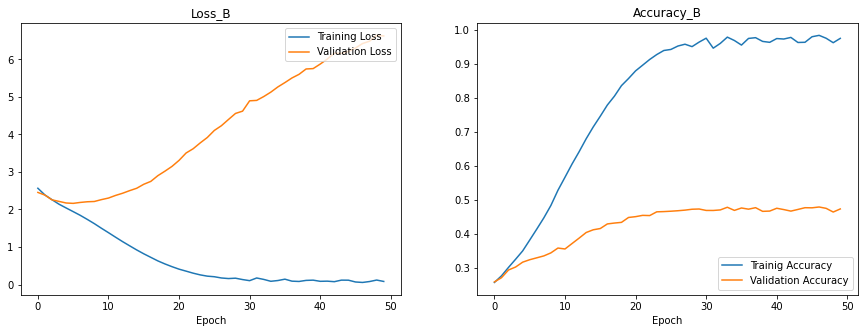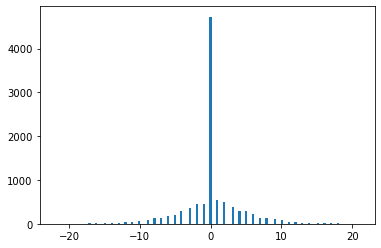# Find prediction errors on validation data¶

```# Import Libraries
from sklearn.model_selection import train_test_split
import tensorflow.keras as keras
from keras import regularizers
import matplotlib.pyplot as plt
import pandas as pd
import numpy as np
```
```# Import Data
path_B = 'data_random.csv' # change to _random
df_B[df_B.no % 2==1] = df_B[df_B.no % 2==1] + 1
```
```# Create Training and Validation Data (B)
X_train_B, X_test_B, y_train_B, y_test_B = train_test_split(
df_B.drop("no", axis=1).astype('float64'),
df_B["no"]//2,
test_size=0.2, random_state=42)

X_train_B, X_test_B, y_train_B, y_test_B = np.asarray(X_train_B), np.asarray(X_test_B), np.asarray(y_train_B), np.asarray(y_test_B)
print(f"There a {len(X_train_B)} training samples and {len(X_test_B)} test samples.")
```
```There a 40000 training samples and 10000 test samples.
```
```# Model Set Up
model_B = keras.Sequential([
keras.layers.Dense(units=1000, activation='relu', input_shape=(18,)),
keras.layers.Dense(units=1000, activation='relu'),
keras.layers.Dense(units=93, activation='softmax')
])

sce = keras.losses.SparseCategoricalCrossentropy()
```
```# Train Model
history_B = model_B.fit(
X_train_B,
y_train_B,
verbose=0,
epochs=50,
batch_size=64,
validation_data=(
X_test_B,
y_test_B
)
)
```
```# Plot Training History
fig, axs = plt.subplots(1, 2, figsize=(15, 5))
axs.plot(history_B.history['loss'], label="Training Loss")
axs.plot(history_B.history['val_loss'], label="Validation Loss")
axs.set_title("Loss_B")
axs.legend(loc="upper right")
axs.plot(history_B.history['accuracy'], label="Trainig Accuracy")
axs.plot(history_B.history['val_accuracy'], label="Validation Accuracy")
axs.legend(loc="lower right")
axs.set_title("Accuracy_B")
axs.sharex(axs)

for ax in axs.flat:
ax.set(xlabel='Epoch')
fig.show()
``````# Print Accuracies
print(f"Validation Accuracy of model_B: {history_B.history['val_accuracy'][-1]}")
print(f"Training Accuracy of model_B : {history_B.history['accuracy'][-1]}")
```
```Validation Accuracy of model_B: 0.47269999980926514
Training Accuracy of model_B : 0.9741500020027161
```
```# Analyse Error Distribution on Testing Data
df = pd.DataFrame(X_test_B)
prediction = np.argmax(model_B.predict(df), axis=1)
delta = y_test_B - prediction
delta_dist = {val: 0 for val in sorted(set(delta))}
for val in delta:
delta_dist[val] += 1

delta_hist = [key for key, val in delta_dist.items() for _ in range(val)]
fig = plt.hist(delta_hist, bins=136)
plt.show()
```
```313/313 [==============================] - 1s 2ms/step
```